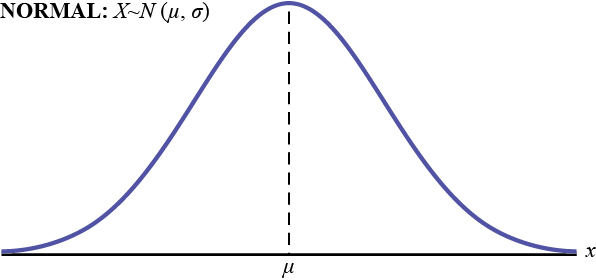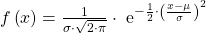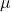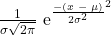The Normal Distribution

# 29 Introduction

If you ask enough people about their shoe size, you will find that your graphed data is shaped like a bell curve and can be described as normally distributed. (credit: Ömer Ünlϋ)The normal probability density function, a continuous distribution, is the most important of all the distributions. It is widely used and even more widely abused. Its graph is bell-shaped. You see the bell curve in almost all disciplines. Some of these include psychology, business, economics, the sciences, nursing, and, of course, mathematics. Some of your instructors may use the normal distribution to help determine your grade. Most IQ scores are normally distributed. Often real-estate prices fit a normal distribution.

The normal distribution is extremely important, but it cannot be applied to everything in the real world. Remember here that we are still talking about the distribution of population data. This is a discussion of probability and thus it is the population data that may be normally distributed, and if it is, then this is how we can find probabilities of specific events just as we did for population data that may be binomially distributed or Poisson distributed. This caution is here because in the next chapter we will see that the normal distribution describes something very different from raw data and forms the foundation of inferential statistics.

The normal distribution has two parameters (two numerical descriptive measures): the mean (μ) and the standard deviation (σ). If X is a quantity to be measured that has a normal distribution with mean (μ) and standard deviation (σ), we designate this by writing the following formula of the normal probability density function:The probability density function is a rather complicated function. Do not memorize it. It is not necessary.The curve is symmetric about a vertical line drawn through the mean, μ. The mean is the same as the median, which is the same as the mode, because the graph is symmetric about μ. As the notation indicates, the normal distribution depends only on the mean and the standard deviation. Note that this is unlike several probability density functions we have already studied, such as the Poisson, where the mean is equal toand the standard deviation simply the square root of the mean, or the binomial, where p is used to determine both the mean and standard deviation. Since the area under the curve must equal one, a change in the standard deviation, σ, causes a change in the shape of the normal curve; the curve becomes fatter and wider or skinnier and taller depending on σ. A change in μ causes the graph to shift to the left or right. This means there are an infinite number of normal probability distributions. One of special interest is called the standard normal distribution.

### Formula Review

XN(μ, σ)

μ = the meanσ = the standard deviation

### Key Terms

Normal Distribution
a continuous random variable (RV) with pdf f(x) =, where μ is the mean of the distribution and σ is the standard deviation; notation: X ~ N(μ, σ). If μ = 0 and σ = 1, the RV, Z, is called the standard normal distribution.